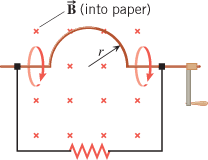# Simple magnetic flux question1. A loop of wire has the shape shown in the drawing. The top part of the wire is bent into a semicircle of radius . The normal to the plane of the loop is parallel to a constant magnetic field of magnitude 0.75 T. What is the change in the magnetic flux that passes through the loop when, starting with the position shown in the drawing, the semicircle is rotated through half a revolution?

## Homework Equations

A = Pi(radius^2) / 2 = (Pi)(0.2^2)/2 = 0.0628
Flux = BA

## The Attempt at a Solution

Change in flux = final flux - original flux = BA[cos(final angle) - cos(initial angle)]
Change in flux = 0.75(0.0628)[cos180-cos0] = -0.094 Tm^2

But I am unsure how why the final angle is 180 such that you get a flux that is negative as calculated below. I guess I just cannot conceptualize WHY the the angle is 180 degrees when the wire goes through half of a revolution. I mean, when it goes through this half-revolution, the plane is still parallel to the screen of the computer. Thus, isn't the angle between plane of the computer screen and the magnetic field (which goes into the screen of the computer) still 0?

Last edited:

Doc Al
Mentor
The attachment isn't viewable. Can you try to attach it again.

Yes here it is thank you!

Doc Al
Mentor
But I am unsure how why the final angle is 180 such that you get a flux that is negative as calculated below. I guess I just cannot conceptualize WHY the the angle is 180 degrees when the wire goes through half of a revolution. I mean, when it goes through this half-revolution, the plane is still parallel to the screen of the computer. Thus, isn't the angle between plane of the computer screen and the magnetic field (which goes into the screen of the computer) still 0?
The change in flux is due to the change in the area of the loop, not in any change in angle. Originally, the area of the loop is a rectangle plus a semicircle. But when the semicircle flips over, the area is now the rectangle minus a semicircle.

Wow - I pay for chegg to understand solutions to problems. It misled me to believe that it had to do with angle.

Thank you so much Doc Al. You always pull through!